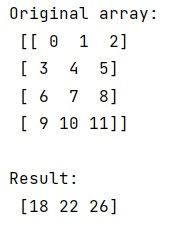# How to calculate the sum of all columns of a 2D numpy array (efficiently)?

Learn, how to calculate the sum of all columns of a 2D numpy array (efficiently) in Python?
Submitted by Pranit Sharma, on January 15, 2023

NumPy is an abbreviated form of Numerical Python. It is used for different types of scientific operations in python. Numpy is a vast library in python which is used for almost every kind of scientific or mathematical operation. It is itself an array which is a collection of various methods and functions for processing the arrays.

## Calculating the sum of all columns of a 2D NumPy array

Suppose that we are given a 2D NumPy array containing some rows and columns and we need to find an efficient way to generate a 1D array that contains the sum of all columns.

For this purpose, we will use the NumPy sum method while paying some extra attention to the axis argument, to sum over the columns we will use the argument axis=0.

Let us understand with the help of an example,

## Python code to calculate the sum of all columns of a 2D numpy array

```# Import numpy
import numpy as np

# Creating a numpy array
arr = np.arange(12).reshape(4,3)

# Display original array
print("Original array:\n",arr,"\n")

# Finding the sum of each column
res = np.sum(arr,axis=0)

print("Result:\n",res)
```

Output:What's New (MCQs)

Top Interview Coding Problems/Challenges!

IncludeHelp's Blogs

Languages: » C » C++ » C++ STL » Java » Data Structure » C#.Net » Android » Kotlin » SQL
Web Technologies: » PHP » Python » JavaScript » CSS » Ajax » Node.js » Web programming/HTML
Solved programs: » C » C++ » DS » Java » C#
Aptitude que. & ans.: » C » C++ » Java » DBMS
Interview que. & ans.: » C » Embedded C » Java » SEO » HR
CS Subjects: » CS Basics » O.S. » Networks » DBMS » Embedded Systems » Cloud Computing
» Machine learning » CS Organizations » Linux » DOS
More: » Articles » Puzzles » News/Updates